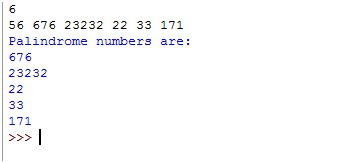# Python | Program to print Palindrome numbers from the given list

In this, we have a list of numbers from that list we have to print only palindrome numbers present in that list, palindrome numbers are numbers, on reversing which number remains the same.
Submitted by Anamika Gupta, on July 17, 2018

First few palindrome numbers are 0, 1 , 2 , 3 , 4 , 5 , 6 , 7 , 8 , 9 , 11 , 22 , 33 , 44 , 55 , 66 , 77 , 88 , 99 , 101 , 111 , 121 , ... and so on.

Input format: Given a number n, size of list then next line contains space separated n numbers.

Logic: We will simply convert the number into string and then using reversed(string) predefined function in python ,we will check whether the reversed string is same as the number or not.

Program:

```# Give size of list
n=int(input())

# Give list of numbers having size n
l=list(map(int,input().strip().split(' ')))

print("Palindrome numbers are:")
# check through the list to check
# number is palindrome or not
for i in l:
num=str(i)
if("".join(reversed(num))==num):
print(i)
```

OutputPreparation